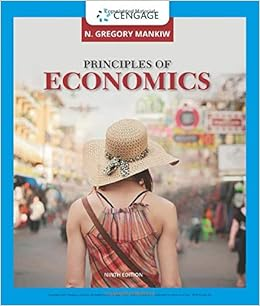# A firm hires labor up to the point where the a real

• Test Prep
• 23
• 88% (8) 7 out of 8 people found this document helpful

This preview shows page 12 - 14 out of 23 pages.

MACRO ECONOMICS STUDY GUIDE

58 )   The demand for labor reflects the point that the
A ) lower the real wage rate , the greater the quantity of labor demanded .
B ) higher the real wage rate , the greater the quantity of labor demanded .
C ) real wage rate does not effect the quantity demanded of labor .
D ) nominal wage rate and not the real wage rate determines the quantity of labor demanded .
59 )   The demand for labor curve is
60 )   A firm ’s demand for labor depends on the
61 )   The quantity of labor demanded by a firm depends upon
62 )   A firm hires labor up to the point where the
A ) real wage rate equals the nominal wage rate .
B ) additional hour of labor produces extra output that equals the real wage rate .
C ) additional hour of labor produces extra output that equals the nominal wage rate .
D ) firm can sell the extra output .
63 )   As long as an additional worker hired by a firm produces
64 )   Hershey Chocolate Factory pays a money wage rate equal to \$ 22.50 per hour and sells it s candy b ars for \$ 1.25 each . Hershey Chocolate Factory should hire labor until an additional unit of labor p roduces ____ candy bars an hour .
65 )   Loafing the Day Away bread company sells fresh bread for \$ 4.00 a loaf . If the last worker hired a dds an additional 7 loaves of bread per hour , then the money wage rate is ____ per hour .
66 )   The Bubby Gum factory produces bubble gum . Joanne is one of the employees , and she produce 10 packs of bubble gum per hour . Joanne ’s money wage rate is \$ 12 per hour . Based on this inform ation , the Bubby Gum company should
A ) keep Joanne because she creates a profit for the firm .
B ) fire Joanne because she creates a loss for the firm .
C ) decrease Joanne ’s wage rate because she is paid too much .
D ) None of the above answers are correct because more information is needed to decide what to do with Joanne .
Answer:  D )   None of the above answers are correct because more information is needed to decide what to do with Joanne .
67 )   The Bubby Gum factory produces bubble gum . Joanne is one of the employees , and she produces 10 packs of bubble gum per hour . Joanne ’s money wage rate is \$ 12 per hour . If a packet of bubble gum sells for \$ 1.00 , then
68 )   To maximize profits , firms hire labor as long as
69 )   An increase in the wage rate ____ the quantity of labor supplied and __ __ the quantity of labor de manded .
70 )   For a household , the opportunity cost of not working is the
A ) nominal wage rate .
B ) real wage rate .
C ) cost of living .
D ) None of the above answers is correct .
Answer:  B )   real wage rate .
##### We have textbook solutions for you!
The document you are viewing contains questions related to this textbook.The document you are viewing contains questions related to this textbook.
Chapter 18 / Exercise 5
Principles of Economics
MankiwExpert Verified
62) A firm hires labor up to the point where the A) real wage rate equals the nominal wage rate. B) additional hour of labor produces extra output that equals the real wage rate. C) additional hour of labor produces extra output that equals the nominal wage rate. D) firm can sell the extra output.
63) As long as an additional worker hired by a firm produces
64) Hershey Chocolate Factory pays a money wage rate equal to \$22.50 per hour and sells its candy b ars for \$1.25 each. Hershey Chocolate Factory should hire labor until an additional unit of labor p roduces ____ candy bars an hour.
##### We have textbook solutions for you!
The document you are viewing contains questions related to this textbook.The document you are viewing contains questions related to this textbook.
Chapter 18 / Exercise 5
Principles of Economics
MankiwExpert Verified
65) Loafing the Day Away bread company sells fresh bread for \$4.00 a loaf. If the last worker hired a dds an additional 7 loaves of bread per hour, then the money wage rate is ____ per hour.
66) The Bubby Gum factory produces bubble gum. Joanne is one of the employees, and she produce 10 packs of bubble gum per hour. Joanne’s money wage rate is \$12 per hour. Based on this inform ation, the Bubby Gum company should A) keep Joanne because she creates a profit for the firm. B) fire Joanne because she creates a loss for the firm. C) decrease Joanne’s wage rate because she is paid too much. D) None of the above answers are correct because more information is needed to decide what to do with Joanne.
67) The Bubby Gum factory produces bubble gum. Joanne is one of the employees, and she produces 10 packs of bubble gum per hour. Joanne’s money wage rate is \$12 per hour. If a packet of bubble gum sells for \$1.00, then
68) To maximize profits, firms hire labor as long as
•••